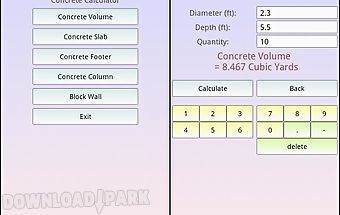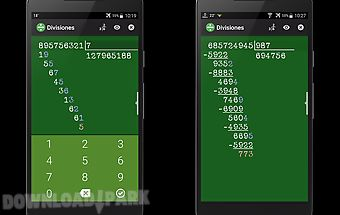# Smart photographic calculator Android App

### Free Apk Files » Other Apps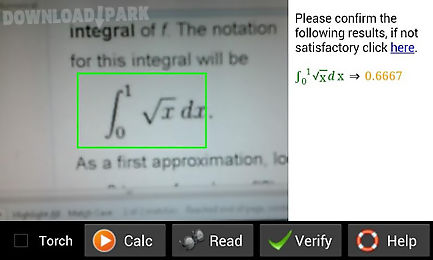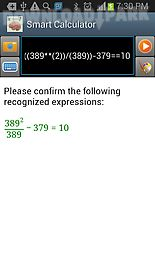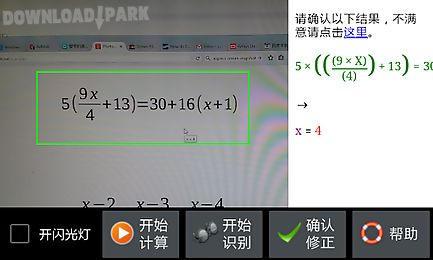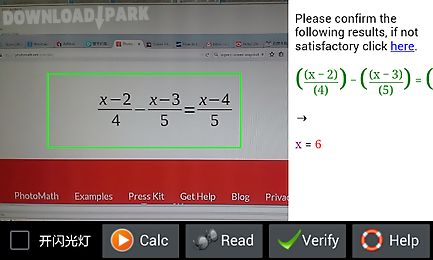The description of Smart photographic calculator: Smart photographic calculator is a powerful tool to recognize (back camera is required) analyze solve and plot mathematical expressions. It supports printed math expression recognition complex number matrix (higher order) integral unit conversion and 2d 3d polar chart plotting. It is based on a powerful math engine mfp programming language. However to make it easy to use user is not allowed to define functions. Smart photographic calculator supports basic arithmetic operations like + mixed and complex fractions and power (80 accuracy) multi variable linear equations (80 accuracy variable name should be x a b c or y) polynomial (80 accuracy variable name should be x a b c or y) (first level) integral (70 accuracy) summation (80 accuracy) and basic matrix calculation (60 accuracy). Operator can be complex values. Note that handwriting math recognition is still in beta stage..

## Similar Apps to Smart photographic calculator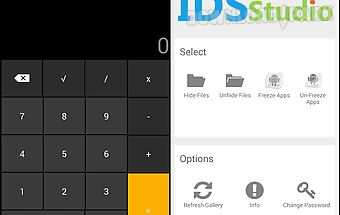Smart hide calculator
smart hide calculator is a fully functional calculator app but with a little twist. Once you enter the password(u get to set it on first start of app and can also be ch..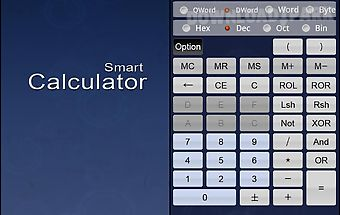Smart scientific calculator
Smart calculator it is all in one! ! Scientific calculator mode. Computer calculator mode. Statistics calculator mode. Sin cos tan sinh cosh tanh log mod exp + 1 int inv..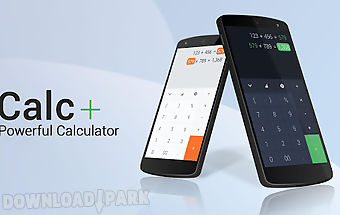Calc+ ★ smart calculator
Your calculator has any features such as: touching to edit number and operator reusing previous calculation result for the next calculation sharing and storing result wi..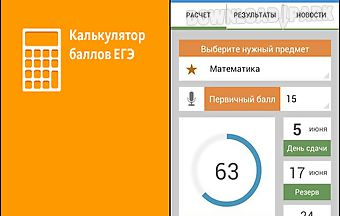Use calculator points
Replace unhandy grade calculation tables with a functional application. Calculate your results maximum fast and precisely. Get only true data corresponding to all educat..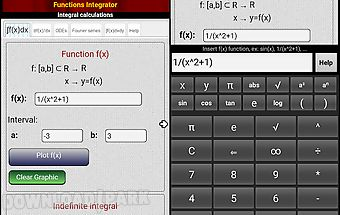Integral calculator
This app works with functions of one and two variables. Integral calculator is designed for students and teachers in maths engineering phisycs and sciences in general. A..Percentages calculator
Simple percentage calculator with big buttons. Ideal for quick calculations. If you like this application you can not miss the full version with more rapid improvements ..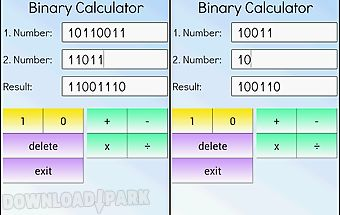Binary calculator
This is a free mathematical calculator which is able to add subtract multiply and divide numbers in binary format (used in computers). Any number can be represented by a..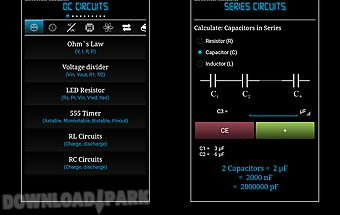Electronics calculator
Electronics calculator is a utility application consisting of various calculators conversions reference tables and basic pocket calculator. Calculators:dc circuits: ohm ..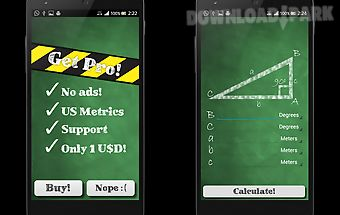Trigonometric calculator
Insert two sides or one angle and one side of a right angled triangle and the trigonometric calculator will do the rest! This is the right angled triangle solver you wer..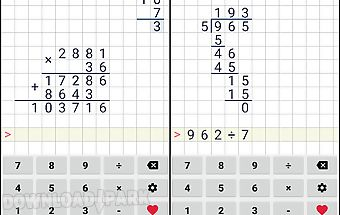Division calculator